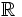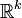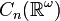# Configuration space of unordered points of a countable-dimensional real vector space

Jump to: navigation, search

## Definition

Let$n$ be a natural number.

Denote by$\R^\omega$ a countable-dimensional real vector space over$\R$; explicitly, it is the space of sequences of real numbers with at most finitely many nonzero entries, under coordinate-wise addition and scalar multiplication. We put a topology on it using the coherent topology arising as the union of finite-dimensional$\R^k$s for initial coordinates.

We can now consider the corresponding configuration space of unordered points. If there are$n$ unordered points, this space, denoted$C_n(\R^\omega)$, is the space of all possible configurations of$n$ distinct unordered points in$\R^\omega$.

## Facts

The configuration space of$n$ unordered points is a path-connected aspherical space and its universal covering space is the corresponding configuration space of ordered points. The fundamental group is the symmetric group of degree$n$. Thus, this space can be viewed as a classifying space for the symmetric group of degree$n$.